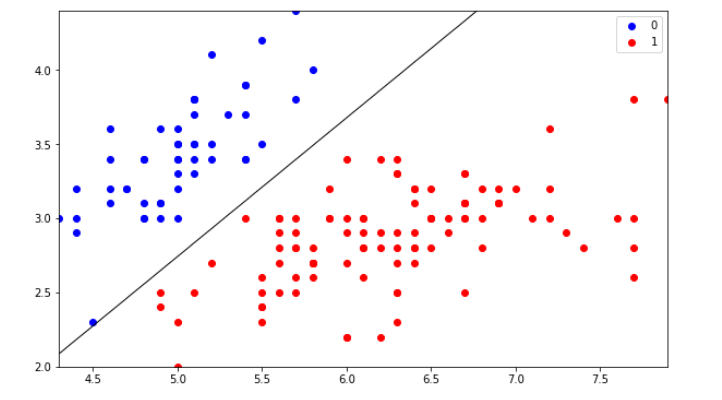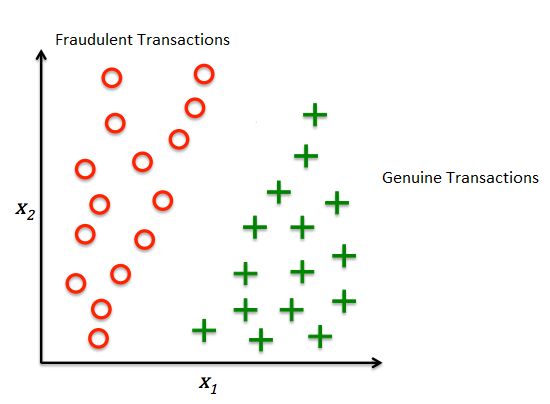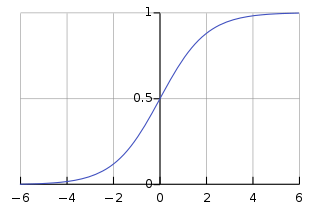# LOGISTIC REGRESSION FROM SCRATCHWelcome to the new machine learning from scratch tutorial. In today’s tutorial, we are going to cover Logistic Regression from scratch. First of all, we would like to thank Bishal Boss who helped us to make this content possible. Now, before we begin, we assume you are already familiar with some of the topics:

• – Classification and Regression in Machine Learning.
• – What is a Binary Classification?
• – Basic Geometry of Line, Plane, and Hyper-Plane in 2D, 3D, and n-D space respectively.
• – What is Maxima & Minima?
• – Loss Function.

INTRODUCTION TO REGRESSION

Regression analysis is to know the nature of the relationship between two or more variables to use it for predicting the most likely value of dependent variables for a given value of independent variables. READ MORE >>

Someone said, “We only learn great things, by questioning them.” So, let’s start with questions and their respective answer. In this blog, we will use LR for Logistic Regression.

#### WHAT IS LOGISTIC REGRESSION?

In simple terms, Logistic Regression is a Classification technique, which is best used for Binary Classification Problems. Logistic regression is used to describe data and to explain the relationship between one dependent binary variable and one or more independent variables.

Let’s say, we have a Binary Classification problem, which has only 2 classes, true or false. Imagine we want to detect whether a credit card transaction is genuine or fraudulent.

• +1 = Genuine
• -1 = Fraudulent

Firstly, let’s try to plot our data points in a 2D space, considering we can visualize 2D better here.#### HOW DO WE DIFFERENTIATE DATA POINTS?

As a human being, if we show this image to a little child with no knowledge of Maths or graphs and ask them to differentiate between the two points, I am pretty much sure that he will use his common sense and draw a line in-between the two points which will differentiate them. That line is called a Decision Boundary, as shown below:

Anything on the left side of the boundary is Fraudulent, and anything on the right side of the boundary is Genuine. This is a common-sense approach.

#### HOW TO BUILD BOUNDARY?

Now, if we look at it from a Geometric perspective, the decision boundary is a simple Line in 2D space. And we know that line also has an equation that can be used to represent it. Now, a line can be represented using two unique variables, “m” and “b”:

In two dimensions, the equation for non-vertical lines is often given in the slope-intercept form: y = mx + b where:

• m is the slope or gradient of the line.
• b is the y-intercept of the line.
• x is the independent variable of the function y = f(x).

If our line passes through the origin, then b = 0, then y = mx

In 3D space we have the equation of Plane as:

Ax + By + Cz = D

If line passes through origin D=0, then z = – (a/c) x + (-b/c) y

Now, this is about 2D and 3D space; in n-D space, we have Hyper-Plane instead of Line or Plane.

#### HOW DO WE REPRESENT HYPERPLANE IN THE EQUATION?

Imagine we have n-dimension in our Hyper-Plane, then each dimension will have its slope value; let’s call them

w1, w2, w3, ….., w

and let the dimensions we represented as

x1, x2, x3, …., x

and let’s have an intercept term as “b.” So, the Hyper-Plane equation holds as:

w1x1 + w2x2 + w3x3 + …. + wnxn + b = 0

We can represent it as:

b + ∑ (wixi) = 0     s.t. (‘i’ ranges from 1 to n)

Now, If we consider wi as a vector and xi as another vector, then we can represent ∑ (wixi) as a vector multiplication of 2 different vectors as WiTXi  where WiT is the transposed vector of values represented using all Wi and Xi   is the vector represented using all values of xi . Also, b here is a scalar value.

Considering all these, Wi is normal to our Hyper-Plane, then only the multiplication would make sense. This means that W is Normal to our Hyper-Plane, and as we all know, Normal is always perpendicular to our surface.

So now, coming back to our model, after all that maths, we conclude that our model needs to learn the Decision Boundary using 2 important things,

1. All the wi values.
2. The intercept b.

For simplicity, let’s consider our plane passes through the origin. Then b=0.

Now, our model needs to only figure out the values of wi , which is normal to our Hyper-Plane. The values in our normal is a vector, which means it is a set of values that needs to be found which best suits our data. So the main task in LR boils down to a simple problem of finding a decision boundary, which is a hyperplane, which is represented using (Wi , b) given a Dataset of (+ve, -ve) points that best separate the points.

Let’s imagine that Wi is derived, so even after that, how are we going to find out the points, whether they are of +1 or -1 class? For that, let’s consider the below Diagram :

So, If you know the basics of ML problems, it can be explained as given a set of Xi, we have to predict Yi. So Yi here belongs to the set {+1, -1}. Now, if we want to calculate the value of di or dj we can do it with the below formula:

di = (WTXi) / ||W||

where W is the normal vector to the out hyperplane, let’s assume that it is a Unit Vector for simplicity. Therefore. ||W|| = 1. Hence, di = WTXi .Similarly, dj = WTXj. Since W and X are on the same side, the Hyper-Plane i.e. on the positive side, hence (WTXi) > 0 and (WTXj) < 0

Basically, it means di belongs to the +1 class, and dj belongs to the -1 class. And this is how we can classify our data points using the Hyper-Plane.

#### HOW DO WE CALCULATE HYPERPLANE?

If you have heard something about optimization problems, our model finds the Hyper-Plane as an optimization problem. Before that, we have to create an optimization equation. Let’s Consider a few cases of yi :

1. Case 1: If a point is Positive, and we predict it as Positive, then
• yi = +1  and  WTXi = +1, then
• yi * (WTXi) > 0
2. Case 2: If a point is Negative, and we predict it as Negative, then
• yi = -1  and  WTXi = -1, then
• yi * (WTXi) > 0
3. Case 3: If a point is Positive, and we predict it as Negative, then
• yi = +1  and  WTXi = -1, then
• yi * (WTXi) < 0
4. Case 4: If a point is Negative, and we predict it as Positive, then
• yi = -1  and  WTXi = +1, then
• yi * (WTXi) < 0

Now, if we look closely, whenever we make a correct prediction, our equation of [yi * (WTXi)] is always positive, irrespective of the cardinality of our data point. Hence, our Optimization equation holds, as such

(Max W) ∑ [yi * (WTXi)] >0 s.t. (i = {1,n} )

Let’s try to understand what the equation has to offer; the equation says that find me a W (the vector normal to our Hyper-Plane) which has a maximum of [yi * (WTXi)] > 0 such that the value of “i” ranges from 1 to n, where “n” is the total number of dimensions we have.

It means for whichever Hyperplane we have the maximum correctly predicted points, we will choose that.

#### HOW DO WE SOLVE THE OPTIMIZATION PROBLEM TO FIND THE OPTIMAL W THAT HAS THE MAX CORRECTLY CLASSIFIED POINT?

Logistic Regression uses a Logistic Function. The logistic function, also called the sigmoid function, is an S-shaped curve that will take any real-valued number and map it into a worth between 0 and 1, but never exactly at those limits.So we use our optimization equation in place of “t”

t = yi * (WTXi) s.t. (i = {1,n} )

And when we solve this sigmoid function, we get another optimization problem, which is computationally easy to solve. The end Optimization equation becomes as follows:

W* = (Argmin W) ∑ logn(1  +  e-t

So, our equation changes from finding a Max to Min; now, we can solve this using an optimizer or a Gradient Descent. To solve this equation, we use something like Gradient Descent; intuitively, it tries to find the minima of a function. In our case, it tries to find the minima of out sigmoid function.

#### HOW DOES IT MINIMIZE A FUNCTION OR FIND MINIMA?

Our Optimizer tries to minimize the loss function of our sigmoid; by loss function I mean it tries to minimize the error made by our model and eventually finds a Hyper-Plane with the lowest error. The loss function has the below equation:

-[y*log(yp) + (i-y)*log(1-yp)]

• y = actual class value of a data point
• yp = predicted class value of data point

And so this is what Logistic Regression is, and that is how we get our best Decision Boundary for classification. In a broader sense, Logistic Regression tries to find the best decision boundary that best separates the data points of different classes.

#### LINEAR REGRESSION VS LOGISTIC REGRESSION

Linear and Logistic Regression are two distinct regression analysis techniques used in different contexts and purposes. Here’s a comparison of these two methods:

###### 1. Purpose:

– Linear Regression: Linear Regression is primarily used for predicting a continuous target variable. It models the relationship between independent variables and the dependent variable as a linear equation. It’s suitable when you want to predict values within a numerical range, such as predicting prices, temperatures, or sales.

– Logistic Regression: Logistic Regression, on the other hand, is used when the dependent variable is binary (two classes, often represented as 0 and 1). It models the probability of an observation belonging to one of the two classes. Logistic Regression is ideal for classification tasks, such as spam detection (spam or not spam), disease diagnosis (disease or no disease), and sentiment analysis (positive or negative sentiment).

###### 2. Output:

– Linear Regression: The output of Linear Regression is a continuous value, and the regression equation is of the form Y = mX + c, where Y is the dependent variable, X is the independent variable, m is the slope (coefficient), and c is the intercept.

– Logistic Regression: The output of Logistic Regression is a probability score between 0 and 1, representing the likelihood of the observation belonging to one of the classes. It typically uses the logistic function (sigmoid) to model this probability.

###### 3. Model Type

– Linear Regression: Linear Regression is a regression model that seeks to find the best-fitting linear relationship between the independent and dependent variables.

– Logistic Regression: Logistic Regression is a classification model. It models the probability of an observation belonging to a particular class and then applies a threshold (usually 0.5) to classify observations into one of the two classes.

###### 4. Assumptions:

– Linear Regression: Linear Regression assumes a linear relationship between variables and that the errors (residuals) are normally distributed and have constant variance.

– Logistic Regression: Logistic Regression assumes that the log odds of the dependent variable being in a particular class is a linear combination of the independent variables. It does not assume normally distributed errors.

###### 5. Evaluation Metrics:

– Linear Regression: Common evaluation metrics include Mean Squared Error (MSE), Root Mean Squared Error (RMSE), and R² Score.

– Logistic Regression: Common evaluation metrics include accuracy, precision, recall, F1-score, and the ROC curve (Receiver Operating Characteristic).

In summary, the choice between Linear Regression and Logistic Regression depends on the nature of the dependent variable and the specific task you want to accomplish. Linear Regression is suitable for predicting continuous values, while Logistic Regression is used for binary classification tasks.

#### CODE FROM SCRATCH

Before that, let’s re-iterate a few key points so the code could make more sense to us:

• # X is a set of data points with m rows and n dimensions.
• # y is a set of class that define a class for every data point from X as +1 or -1
• # z = WTXi
• # W = Set of values for a vector that forms the Normal to our Hyper-Plane.
• # b = Set of scalars of the intercept term, not required if our Hyper-Plane passes through the origin
• # yp = predicted value of Xi,  from the sigmoid function.

Intuitively speaking, our model tries to learn from each iteration using a learning rate and gradient value; once we predict the value using the sigmoid function, we get some values of yp, and then we have y.

We calculate the error, then try to use the error to predict a new set of W values, which we use to repeat the cycle until we finally find the best value possible.

We will work on the Iris dataset in today’s code from scratch. So, let’s dive into the code.

``````%matplotlib inline
import numpy as np
import matplotlib.pyplot as plt
import seaborn as sns
from sklearn import datasets
from sklearn import linear_model

X = iris.data[:, :2]     #we use only 2 class
y = (iris.target != 0) * 1``````

Let’s try to plot and see how our data lies. Whether it can be separated using a decision boundary.

``````plt.scatter(X[y == 0][:, 0], X[y == 0][:, 1], color='b', label='0')
plt.scatter(X[y == 1][:, 0], X[y == 1][:, 1], color='r', label='1')
plt.legend();``````

Let’s train the model by creating a class of it, we will give the Learning rate of 0.1 and the number of iterations as 300000.

``````model = LogisticRegression(learning_rate=0.1, num_iterations=300000)
model.fit(X, y)``````

Let us see how well our prediction works:

``````preds = model.predict(X)
(preds == y).mean()

Output: 1.0``````

#### LOGISTIC REGRESSION FROM SCIKIT LEARN

``````
from sklearn.linear_model import LogisticRegression
from sklearn.metrics import classification_report, confusion_matrix
model = LogisticRegression(solver='liblinear', random_state=0)
model.fit(X, y)
model.predict_proba(X)``````

It’s often useful to visualize the confusion matrix.

``print(classification_report(y, model.predict(X)))``🚀 I'm Diwas Pandey, a Computer Engineer with an unyielding passion for Artificial Intelligence, currently pursuing a Master's in Computer Science at Washington State University, USA. As a dedicated blogger at AIHUBPROJECTS.COM, I share insights into the cutting-edge developments in AI, and as a Freelancer, I leverage my technical expertise to craft innovative solutions. Join me in bridging the gap between technology and healthcare as we shape a brighter future together! 🌍🤖🔬

View all posts by Diwas →

### 14 Comments on “LOGISTIC REGRESSION FROM SCRATCH”

1.resters says:

Perfect piece of work you have done, this website is really cool with excellent info .

2.Magallon says:

Hello, I am so delighted I found your weblog please do keep up the excellent work.

3.Mcclodden says:

Excellent post but I was wanting to know if you could write a litte more on this topic? I’d be very thankful if you could elaborate a little bit further. Thanks!

4.Zanella says:

The article has really peaks my interest.

5.Drollinger says:

You can definitely see your skills in the work you write.

6.Finklestein says:

I appreciate, cause I found exactly what I was looking for. You have ended my four day long hunt! God Bless you man. Have a nice day. Bye

7.Clarendon says:

Nice post. I learn one thing more challenging on completely different blogs everyday.

8.Elbert Bugarin says:

hello there and thanks for your info

9.Jay Patin says:

F*ckin’ amazing things here. I’m very glad to see your article. Thanks a lot and i’m looking forward to contact you. Will you please drop me a mail?

10.web hosting providers says:

I just could not go away your site prior to suggesting
that I actually loved the standard information a person supply in your visitors?
Is gonna be back incessantly in order to investigate cross-check new posts

11. Pingback: Homepage